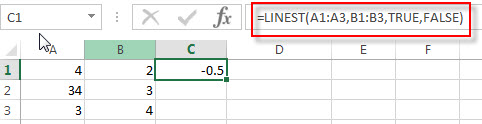# Excel LINEST Function

This post will guide you how to use the LINEST function with syntax and examples in Microsoft excel.

### Description

The Excel LINEST function calculates the statistics for a line by using the “least squares” method to calculate a straight line that best fits your data, and then returns an array that describes the line. So you can use the LINEST function to get the statistical information on the line of best fit based on the existing y-values and x-values in Excel.

The LINEST function will use the following line equation:

```y = mx + b
y = m1x1 + m2x2 + ... + b```

The LINEST function is a build-in function in Microsoft Excel and it is categorized as a Statistical Function.

The LINEST function is available in Excel 2016, Excel 2013, Excel 2010, Excel 2007, Excel 2003, Excel XP, Excel 2000, Excel 2011 for Mac.

### Syntax

The syntax of the LINEST function is as below:

`=LINEST (known_y's, known_x's, [const], [stats])`

Where the LINEST function arguments are:

• known_y’s -This is a required argument.  An array of known y-values from the line equation.
• known_x’s – This is an optional argument.  An array of known x-values from the line equation.
• const – This is an optional argument.  A logical values used to determine whether the constant b is equal to 0. And If the Const argument is equal to TRUE or omitted, the Constant b is calculated. Otherwise, the constant b is set to 0.
• Stats – This is an optional argument.  A logical value used to determine whether the function need to return additional regression statistics. If this argument is TRUE, the LINEST function will return additional regression statistics, otherwise, The LINEST function will return only the m-coefficients and the constant b.

### Excel LINEST Function Examples

The below examples will show you how to use Excel LINEST Function to calculate the statistical information on the line of best fit you data.

#1 to calculate the statistical for a straight line through the existing y-values and x-values, using the following formula:

`=LINEST(A1:A3,B1:B3,TRUE,FALSE)`